# peak- to-average

## English translation: The ratio of the maximum power to the medium power

 13:14 Nov 13, 2020
English language (monolingual) [PRO]
Tech/Engineering - Physics
 English term or phrase: peak- to-average Looking at RZ pulse shape, it makes no difference whether the serial or the par- allel transmitter is used because power is decreased during the symbol transitions and the signal shapes are nearly identical. For NRZ, however, the use of the serial transmitter leads to a worse OSNR performance. This is caused by the smaller peak- to-average power ratio and the higher chirp which results in larger distortions at the optical receiver filter. When comparing the decision techniques, arg-decision shows a better performance than IQ-decision, since the thresholds are optimally placed be- tween the symbols for arg-decision, whereas the distances between the thresholds and the symbols are smaller for IQ-decision. The tendencies shown here for 8DPSK are also valid for other formats.
 Ангелина
 Selected answer:The ratio of the maximum power to the medium power Explanation:The “peak to average power” level, usually expressed in dB, is the power level of the highest instantaneous power compared to the average power level. A PAR of 1, or 0 dB, means the signal is of constant power, so the peak power is equal to the average power.
Selected response from:

Amir Akbarpour Reihani
Local time: 20:56
 Selected automatically based on peer agreement.4 KudoZ points were awarded for this answer

SUMMARY OF ALL EXPLANATIONS PROVIDED
4 +4The ratio of the maximum power to the medium power
 Amir Akbarpour Reihani3 +2peak-to-average power ratio, PAPR
 Neil Ashby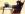4the highest recording (peak) compared to the average recording
 Bridgette Mitchell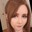13 mins   confidence:peer agreement (net): +4
The ratio of the maximum power to the medium power

Explanation:
The “peak to average power” level, usually expressed in dB, is the power level of the highest instantaneous power compared to the average power level. A PAR of 1, or 0 dB, means the signal is of constant power, so the peak power is equal to the average power.

https://www.sciencedirect.com/topics/engineering/peak-to-average-ratio#:~:text=17.11%20Peak%20to%20Average%20Ratio&text=The%20%E2%80%9Cpeak%
 Amir Akbarpour ReihaniLocal time: 20:56Native speaker of:Farsi (Persian),Turkish,Persian (Farsi)PRO pts in category: 4Selected automatically based on peer agreement.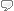Peer comments on this answer (and responses from the answerer)
agree
 3 mins
-> Thank you!

agree  philgoddard: Though it's mean or median, I'm not sure which, rather than medium.
 6 mins
-> Yes, Maybe I should use "mean": https://gymaware.zendesk.com/hc/en-us/articles/115001148431-...

agree
 8 mins
-> Thank you!

agree
 2 hrs
-> Thank you!

19 mins   confidence:peer agreement (net): +2
peak-to-average
peak-to-average power ratio, PAPR

Explanation:
I'm not really sure what your doubt or question is because you haven't posed one, but I found this in two seconds:

What is PAPR (Peak to average power ratio), Why it matters to Power Amplifier ?
The PAPR is the relation between the maximum power of a sample in a given OFDM transmit symbol divided by the average power of that OFDM symbol. In simple terms, PAPR is the ratio of peak power to the average power of a signal. It is expressed in the units of dB.
http://www.techplayon.com/papr-peak-average-power-ratio-matt...

Crest factor is a parameter of a waveform, such as alternating current or sound, showing the ratio of peak values to the effective value. In other words, crest factor indicates how extreme the peaks are in a waveform. Crest factor 1 indicates no peaks, such as direct current or a square wave. Higher crest factors indicate peaks, for example sound waves tend to have high crest factors.

Crest factor is the peak amplitude of the waveform divided by the RMS value of the waveform. This is equivalent to the ratio of the L∞ norm to the L2 norm of the function of the waveform:

C = | x p e a k | x r m s = ‖ x ‖ ∞ ‖ x ‖ 2 {\displaystyle C={\frac {|x_{\mathrm {peak} }|}{x_{\mathrm {rms} }}}={\frac {\|x\|_{\infty }}{\|x\|_{2}}}} {\displaystyle C={\frac {|x_{\mathrm {peak} }|}{x_{\mathrm {rms} }}}={\frac {\|x\|_{\infty }}{\|x\|_{2}}}}
C d B = 20 log 10 ⁡ ( | x p e a k | x r m s ) . {\displaystyle {C}_{\mathrm {dB} }=20\log _{10}\left({|x_{\mathrm {peak} }| \over x_{\mathrm {rms} }}\right).} {\displaystyle {C}_{\mathrm {dB} }=20\log _{10}\left({|x_{\mathrm {peak} }| \over x_{\mathrm {rms} }}\right).}

The peak-to-average power ratio (PAPR) is the peak amplitude squared (giving the peak power) divided by the RMS value squared (giving the average power). It is the square of the crest factor:

P A P R = | x p e a k | 2 x r m s 2 = C 2 {\displaystyle {\mathit {PAPR}}={\frac {{|x_{\mathrm {peak} }|}^{2}}{{x_{\mathrm {rms} }}^{2}}}=C^{2}} {\displaystyle {\mathit {PAPR}}={\frac {{|x_{\mathrm {peak} }|}^{2}}{{x_{\mathrm {rms} }}^{2}}}=C^{2}}
P A P R d B = 10 log 10 ⁡ | x p e a k | 2 x r m s 2 = C d B . {\displaystyle {\mathit {PAPR}}_{\mathrm {dB} }=10\log _{10}{\frac {{|x_{\mathrm {peak} }|}^{2}}{{x_{\mathrm {rms} }}^{2}}}=C_{\mathrm {dB} }.} {\displaystyle {\mathit {PAPR}}_{\mathrm {dB} }=10\log _{10}{\frac {{|x_{\mathrm {peak} }|}^{2}}{{x_{\mathrm {rms} }}^{2}}}=C_{\mathrm {dB} }.}

When expressed in decibels, crest factor and PAPR are equivalent, due to the way decibels are calculated for power ratios vs amplitude ratios.

Crest factor and PAPR are therefore dimensionless quantities. While the crest factor is defined as a positive real number, in commercial products it is also commonly stated as the ratio of two whole numbers, e.g., 2:1. The PAPR is most used in signal processing applications. As it is a power ratio, it is normally expressed in decibels (dB). The crest factor of the test signal is a fairly important issue in loudspeaker testing standards; in this context it is usually expressed in dB.
https://en.wikipedia.org/wiki/Crest_factor

 Neil AshbySpainLocal time: 18:26Works in fieldNative speaker of: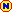English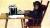Peer comments on this answer (and responses from the answerer)
agree  Yvonne Gallagher: also not really sure what the doubt or question is but very easily found
 12 mins
-> Thanks Yvonne.

agree
 2 hrs

22 mins   confidence:the highest recording (peak) compared to the average recording

Explanation:
For example, if you recorded the sound of a song in decibels, and there is one instance of 89 decibels, but on average the song is around 50 decibels. In this case, 89 is the peak, and 50 is the average. It is the difference between the average recording and the highest recording.

Please find point 17.11 Peak to Average Ratio in the article linked below.

https://www.sciencedirect.com/topics/engineering/peak-to-average-ratio#:~:text=17.11%20Peak%20to%20Average%20Ratio&text=The%20%E2%80%9Cpeak%
 Bridgette MitchellUnited KingdomLocal time: 17:26Native speaker of:EnglishLogin or register (free and only takes a few minutes) to participate in this question.

You will also have access to many other tools and opportunities designed for those who have language-related jobs (or are passionate about them). Participation is free and the site has a strict confidentiality policy.

## KudoZ™ translation help

The KudoZ network provides a framework for translators and others to assist each other with translations or explanations of terms and short phrases.